Previous Page | Next Page

 The MCMC Procedure

## Example 52.3 Generalized Linear Models

This example discusses two examples of fitting generalized linear models (GLM) with PROC MCMC. One uses a logistic regression model and one uses a Poisson regression model. The logistic examples use both a diffuse prior and a Jeffreys’ prior on the regression coefficients. You can also use the BAYES statement in PROC GENMOD. See Chapter 37, The GENMOD Procedure.

### Logistic Regression Model with a Diffuse Prior

The following statements create a SAS data set with measurements of the number of deaths, y, among n beetles that have been exposed to an environmental contaminant x:

```title 'Logistic Regression Model with a Diffuse Prior';
data beetles;
input n y x @@;
datalines;
6  0  25.7   8  2  35.9   5  2  32.9   7  7  50.4   6  0  28.3
7  2  32.3   5  1  33.2   8  3  40.9   6  0  36.5   6  1  36.5
6  6  49.6   6  3  39.8   6  4  43.6   6  1  34.1   7  1  37.4
8  2  35.2   6  6  51.3   5  3  42.5   7  0  31.3   3  2  40.6
;
```

You can model the data points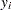with a binomial distribution: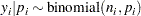where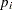is the success probability and links to the regression covariate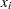through a logit transformation: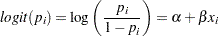The priors onandare both diffuse normal: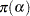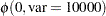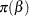These statements fit a logistic regression with PROC MCMC:

```ods graphics on;
proc mcmc data=beetles ntu=1000 nmc=20000 nthin=2 propcov=quanew
diag=(mcse ess) outpost=beetleout seed=246810;
ods select PostSummaries PostIntervals mcse ess TADpanel;
parms (alpha  beta) 0;
prior alpha beta ~ normal(0, var = 10000);
p = logistic(alpha + beta*x);
model y ~ binomial(n,p);
run;
```

The key statement in the program is the assignment to p that calculates the probability of death. The SAS function LOGISTIC does the proper transformation. The MODEL statement specifies that the response variable, y, is binomially distributed with parameters n (from the input data set) and p. The summary statistics table, interval statistics table, the Monte Carlos standard error table, and the effective sample sizes table are shown in Output 52.3.1.

Output 52.3.1 MCMC Results
 Logistic Regression Model with a Diffuse Prior

The MCMC Procedure

Posterior Summaries
Parameter N Mean Standard
Deviation
Percentiles
25% 50% 75%
alpha 10000 -11.7707 2.0997 -13.1243 -11.6683 -10.3003
beta 10000 0.2920 0.0542 0.2537 0.2889 0.3268

Posterior Intervals
Parameter Alpha Equal-Tail Interval HPD Interval
alpha 0.050 -16.3332 -7.9675 -15.8822 -7.6673
beta 0.050 0.1951 0.4087 0.1901 0.4027

 Logistic Regression Model with a Diffuse Prior

The MCMC Procedure

Monte Carlo Standard Errors
Parameter MCSE Standard
Deviation
MCSE/SD
alpha 0.0422 2.0997 0.0201
beta 0.00110 0.0542 0.0203

Effective Sample Sizes
Parameter ESS Autocorrelation
Time
Efficiency
alpha 2470.1 4.0484 0.2470
beta 2435.4 4.1060 0.2435

The summary statistics table shows that the sample mean of the output chain for the parameter alpha is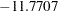. This is an estimate of the mean of the marginal posterior distribution for the intercept parameter alpha. The estimated posterior standard deviation for alpha is 2.0997. The two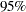credible intervals for alpha are both negative, which indicates with very high probability that the intercept term is negative. On the other hand, you observe a positive effect on the regression coefficient beta. Exposure to the environment contaminant increases the probability of death.

The Monte Carlo standard errors of each parameter are significantly small relative to the posterior standard deviations. A small MCSE/SD ratio indicates that the Markov chain has stabilized and the mean estimates do not vary much over time. Note that the precision in the parameter estimates increases with the square of the MCMC sample size, so if you want to double the precision, you must quadruple the MCMC sample size.

MCMC chains do not produce independent samples. Each sample point depends on the point before it. In this case, the correlation time estimate, read from the effective sample sizes table, is roughly 4. This means that it takes four observations from the MCMC output to make inferences about alpha with the same precision that you would get from using an independent sample. The effective sample size of 2470 reflects this loss of efficiency. The coefficient beta has similar efficiency. You can often observe that some parameters have significantly better mixing (better efficiency) than others, even in a single Markov chain run.

Output 52.3.2 Plots for Parameters in the Logistic Regression Example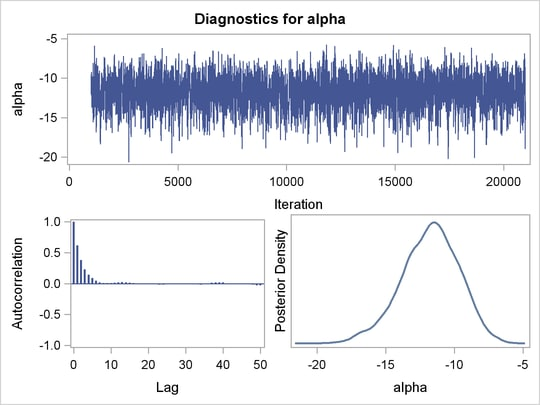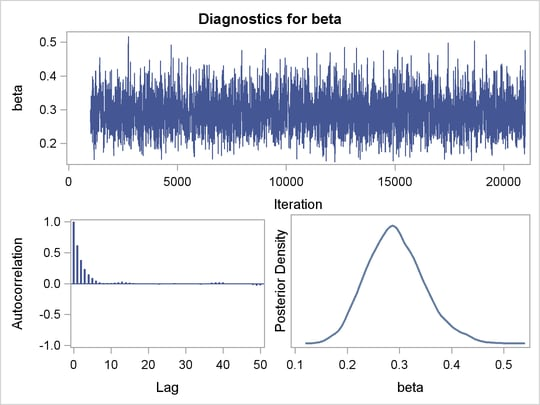Trace plots and autocorrelation plots of the posterior samples are shown in Output 52.3.2. Convergence looks good in both parameters; there is good mixing in the trace plot and quick drop-off in the ACF plot.

One advantage of Bayesian methods is the ability to directly answer scientific questions. In this example, you might want to find out the posterior probability that the environmental contaminant increases the probability of death—that is,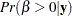. This can be estimated using the following steps:

```proc format;
value betafmt low-0 = 'beta <= 0' 0<-high = 'beta > 0';
run;

proc freq data=beetleout;
tables beta /nocum;
format beta betafmt.;
run;
```

Output 52.3.3 Frequency Counts
 Logistic Regression Model with a Diffuse Prior

The FREQ Procedure

beta Frequency Percent
beta > 0 10000 100.00

All of the simulated values forare greater than zero, so the sample estimate of the posterior probability that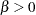is 100%. The evidence overwhelmingly supports the hypothesis that increased levels of the environmental contaminant increase the probability of death.

If you are interested in making inference based on any quantities that are transformations of the random variables, you can either do it directly in PROC MCMC or by using the DATA step after you run the simulation. Transformations sometimes can make parameter inference quite formidable using direct analytical methods, but with simulated chains, it is easy to compute chains for any set of parameters. Suppose that you are interested in the lethal dose and want to estimate the level of the covariate x that corresponds to a probability of death,. Abbreviate this quantity as ldp. In other words, you want to solve the logit transformation with a fixed value. The lethal dose is as follows: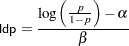You can obtain an estimate of any ldp by using the posterior mean estimates forand. For example, lp95, which corresponds to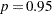, is calculated as follows: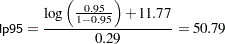where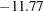and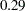are the posterior mean estimates ofand, respectively, and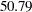is the estimated lethal dose that leads to adeath rate.

While it is easy to obtain the point estimates, it is harder to estimate other posterior quantities, such as the standard deviation directly. However, with PROC MCMC, you can trivially get estimates of any posterior quantities of lp95. Consider the following program in PROC MCMC:

```proc mcmc data=beetles ntu=1000 nmc=20000 nthin=2 propcov=quanew
outpost=beetleout seed=246810 plot=density
monitor=(pi30 ld05 ld50 ld95);
ods select PostSummaries PostIntervals densitypanel;
parms (alpha  beta) 0;
begincnst;
c1 = log(0.05 / 0.95);
c2 = -c1;
endcnst;

beginnodata;
prior alpha beta ~ normal(0, var = 10000);
pi30 = logistic(alpha + beta*30);
ld05 = (c1 - alpha) / beta;
ld50 = - alpha / beta;
ld95 = (c2 - alpha) / beta;
endnodata;
pi = logistic(alpha + beta*x);
model y ~ binomial(n,pi);
run;
ods graphics off;
```

The program estimates four additional posterior quantities. The three lpd quantities, ld05, ld50, and ld95, are the three levels of the covariate that kills 5%, 50%, and 95% of the population, respectively. The predicted probability when the covariate x takes the value of 30 is pi30. The MONITOR= option selects the quantities of interest. The PLOTS= option selects kernel density plots as the only ODS graphical output, excluding the trace plot and autocorrelation plot.

Programming statements between the BEGINCNST and ENDCNST statements define two constants. These statements are executed once at the beginning of the simulation. The programming statements between the BEGINNODATA and ENDNODATA statements evaluate the quantities of interest. The symbols, pi30, ld05, ld50, and ld95, are functions of the parameters alpha and beta only. Hence, they should not be processed at the observation level and should be included in the BEGINNODATA and ENDNODATA statements. Output 52.3.4 lists the posterior summary and Output 52.3.5 shows the density plots of these posterior quantities.

Output 52.3.4 PROC MCMC Results
 Logistic Regression Model with a Diffuse Prior

The MCMC Procedure

Posterior Summaries
Parameter N Mean Standard
Deviation
Percentiles
25% 50% 75%
pi30 10000 0.0524 0.0253 0.0340 0.0477 0.0662
ld05 10000 29.9281 1.8814 28.8430 30.1727 31.2563
ld50 10000 40.3745 0.9377 39.7271 40.3165 40.9612
ld95 10000 50.8210 2.5353 49.0372 50.5157 52.3100

Posterior Intervals
Parameter Alpha Equal-Tail Interval HPD Interval
pi30 0.050 0.0161 0.1133 0.0109 0.1008
ld05 0.050 25.6409 32.9660 26.2193 33.2774
ld50 0.050 38.6706 42.3718 38.6194 42.2811
ld95 0.050 46.7180 56.7667 46.3221 55.8774

The posterior mean estimate of lp95 is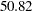, which is close to the estimate ofby using the posterior mean estimates of the parameters. With PROC MCMC, in addition to the mean estimate, you can get the standard deviation, quantiles, and interval estimates at any level of significance.

From the density plots, you can see, for example, that the sample distribution for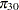is skewed to the right, and almost all of your posterior belief concerningis concentrated in the region between zero and 0.15.

Output 52.3.5 Density Plots of Quantities of Interest in the Logistic Regression Example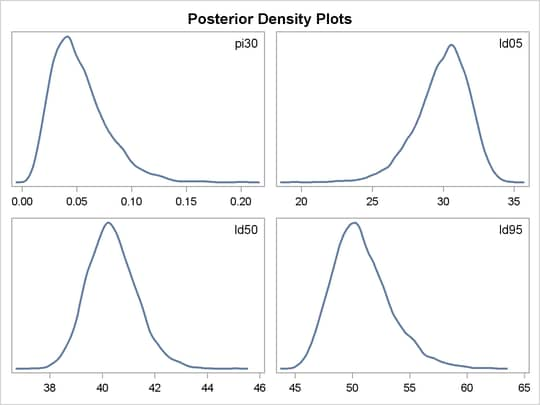It is easy to use the DATA step to calculate these quantities of interest. The following DATA step uses the simulated values ofandto create simulated values from the posterior distributions of ld05, ld50, ld95, and:

```data transout;
set beetleout;
pi30 = logistic(alpha + beta*30);
ld05 = (log(0.05 / 0.95) - alpha) / beta;
ld50 = (log(0.50 / 0.50) - alpha) / beta;
ld95 = (log(0.95 / 0.05) - alpha) / beta;
run;
```

Subsequently, you can use SAS/INSIGHT, or the UNIVARIATE, CAPABILITY, or KDE procedures to analyze the posterior sample. If you want to regenerate the default ODS graphs from PROC MCMC, see Regenerating Diagnostics Plots.

### Logistic Regression Model with Jeffreys’ Prior

A controlled experiment was run to study the effect of the rate and volume of air inspired on a transient reflex vasoconstriction in the skin of the fingers. Thirty-nine tests under various combinations of rate and volume of air inspired were obtained (Finney; 1947). The result of each test is whether or not vasoconstriction occurred. Pregibon (1981) uses this set of data to illustrate the diagnostic measures he proposes for detecting influential observations and to quantify their effects on various aspects of the maximum likelihood fit. The following statements create the data set vaso:

```title 'Logistic Regression Model with Jeffreys Prior';
data vaso;
input vol rate resp @@;
lvol = log(vol);
lrate = log(rate);
ind = _n_;
cnst = 1;
datalines;
3.7  0.825  1  3.5  1.09  1  1.25  2.5   1  0.75  1.5  1
0.8  3.2    1  0.7  3.5   1  0.6   0.75  0  1.1   1.7  0
0.9  0.75   0  0.9  0.45  0  0.8   0.57  0  0.55  2.75 0
0.6  3.0    0  1.4  2.33  1  0.75  3.75  1  2.3  1.64  1
3.2  1.6    1  0.85 1.415 1  1.7   1.06  0  1.8  1.8   1
0.4  2.0    0  0.95 1.36  0  1.35  1.35  0  1.5  1.36  0
1.6  1.78   1  0.6  1.5   0  1.8   1.5   1  0.95 1.9   0
1.9  0.95   1  1.6  0.4   0  2.7   0.75  1  2.35 0.03  0
1.1  1.83   0  1.1  2.2   1  1.2   2.0   1  0.8  3.33  1
0.95 1.9    0  0.75 1.9   0  1.3   1.625 1
;
```

The variable resp represents the outcome of a test. The variable lvol represents the log of the volume of air intake, and the variable lrate represents the log of the rate of air intake. You can model the data by using logistic regression. You can model the response with a binary likelihood: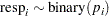with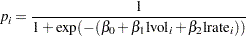Let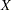be the design matrix in the regression. Jeffreys’ prior for this model is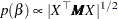where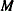is a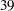bymatrix with off-diagonal elements beingand diagonal elements being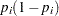. For details on Jeffreys’ prior, see Jeffreys’ Prior. You can use a number of matrix functions, such as the determinant function, in PROC MCMC to construct Jeffreys’ prior. The following statements illustrate how to fit a logistic regression with Jeffreys’ prior:

```/* fitting a logistic regression with Jeffreys' prior */
%let n = 39;
proc mcmc data=vaso nmc=10000 outpost=mcmcout seed=17;
ods select PostSummaries PostIntervals;

array beta beta0 beta1 beta2;
array m[&n, &n];
array x[&n, 3];
array xt[3, &n];
array xtm[3, &n];
array xmx[3, 3];
array p[&n];

parms beta0 1 beta1 1 beta2 1;

begincnst;
x[ind, 1] = 1;
x[ind, 2] = lvol;
x[ind, 3] = lrate;
if (ind eq &n) then do;
call transpose(x, xt);
call zeromatrix(m);
end;
endcnst;

beginnodata;
call mult(x, beta, p);              /* p = x * beta */
do i = 1 to &n;
p[i] = 1 / (1 + exp(-p[i]));     /* p[i] = 1/(1+exp(-x*beta)) */
m[i,i] = p[i] * (1-p[i]);
end;
call mult (xt, m, xtm);             /* xtm = xt * m        */
call mult (xtm, x, xmx);            /* xmx = xtm * x       */
call det (xmx, lp);                 /* lp = det(xmx)       */
lp = 0.5 * log(lp);                 /* lp = -0.5 * log(lp) */
prior beta: ~ general(lp);
endnodata;

model resp ~ bern(p[ind]);
run;
```

The first ARRAY statement defines an array beta with three elements: beta0, beta1, and beta2. The subsequent statements define arrays that are used in the construction of Jeffreys’ prior. These include m (thematrix), x (the design matrix), xt (the transpose of x), and some additional work spaces.

The explanatory variables lvol and lrate are saved in the array x in the BEGINCNST and ENDCNST statements. See BEGINCNST/ENDCNST Statement for details. After all the variables are read into x, you transpose the x matrix and store it to xt. The ZEROMATRIX function call assigns all elements in matrix m the value zero. To avoid redundant calculation, it is best to perform these calculations as the last observation of the data set is processed—that is, when ind is 39.

You calculate Jeffreys’ prior in the BEGINNODATA and ENDNODATA statements. The probability vector p is the product of the design matrix x and parameter vector beta. The diagonal elements in the matrix m are. The expression lp is the logarithm of Jeffreys’ prior. The PRIOR statement assigns lp as the prior for theregression coefficients. The MODEL statement assigns a binary likelihood to resp, with probability p[ind]. The p array is calculated earlier using the matrix function MULT. You use the ind variable to pick out the right probability value for each resp.

Posterior summary statistics are displayed in Output 52.3.6.

Output 52.3.6 PROC MCMC Results, Jeffreys’ prior
 Logistic Regression Model with Jeffreys Prior

The MCMC Procedure

Posterior Summaries
Parameter N Mean Standard
Deviation
Percentiles
25% 50% 75%
beta0 10000 -2.9587 1.3258 -3.8117 -2.7938 -2.0007
beta1 10000 5.2905 1.8193 3.9861 5.1155 6.4145
beta2 10000 4.6889 1.8189 3.3570 4.4914 5.8547

Posterior Intervals
Parameter Alpha Equal-Tail Interval HPD Interval
beta0 0.050 -5.8247 -0.7435 -5.5936 -0.6027
beta1 0.050 2.3001 9.3789 1.8590 8.7222
beta2 0.050 1.6788 8.6643 1.3611 8.2490

You can also use PROC GENMOD to fit the same model by using the following statements:

```proc genmod data=vaso descending;
ods select PostSummaries PostIntervals;
model resp = lvol lrate / d=bin link=logit;
bayes seed=17 coeffprior=jeffreys nmc=20000 thin=2;
run;
```

The MODEL statement indicates that resp is the response variable and lvol and lrate are the covariates. The options in the MODEL statement specify a binary likelihood and a logit link function. The BAYES statement requests Bayesian capability. The SEED=, NMC=, and THIN= arguments work in the same way as in PROC MCMC. The COEFFPRIOR=JEFFREYS option requests Jeffreys’ prior in this analysis.

The PROC GENMOD statements produce Output 52.3.7, with estimates very similar to those reported in Output 52.3.6. Note that you should not expect to see identical output from PROC GENMOD and PROC MCMC, even with the simulation setup and identical random number seed. The two procedures use different sampling algorithms. PROC GENMOD uses the adaptive rejection metropolis algorithm (ARMS) (Gilks and Wild; 1992; Gilks; 2003) while PROC MCMC uses a random walk Metropolis algorithm. The asymptotic answers, which means that you let both procedures run an very long time, would be the same as they both generate samples from the same posterior distribution.

Output 52.3.7 PROC GENMOD Results
 Logistic Regression Model with Jeffreys Prior

The GENMOD Procedure

Bayesian Analysis

Posterior Summaries
Parameter N Mean Standard
Deviation
Percentiles
25% 50% 75%
Intercept 10000 -2.8731 1.3088 -3.6754 -2.7248 -1.9253
lvol 10000 5.1639 1.8087 3.8451 4.9475 6.2613
lrate 10000 4.5501 1.8071 3.2250 4.3564 5.6810

Posterior Intervals
Parameter Alpha Equal-Tail Interval HPD Interval
Intercept 0.050 -5.8246 -0.7271 -5.5774 -0.6060
lvol 0.050 2.1844 9.2297 2.0112 8.9149
lrate 0.050 1.5666 8.6145 1.3155 8.1922

### Poisson Regression

You can use the Poisson distribution to model the distribution of cell counts in a multiway contingency table. Aitkin et al. (1989) have used this method to model insurance claims data. Suppose the following hypothetical insurance claims data are classified by two factors: age group (with two levels) and car type (with three levels). The following statements create the data set:

```title 'Poisson Regression';
data insure;
input n c car \$ age;
ln = log(n);
if car = 'large' then
do car_dummy1=1;
car_dummy2=0;
end;
else if car = 'medium' then
do car_dummy1=0;
car_dummy2=1;
end;
else
do car_dummy1=0;
car_dummy2=0;
end;

datalines;
500   42  small  0
1200  37  medium 0
100    1  large  0
400  101  small  1
500   73  medium 1
300   14  large  1
;
```

The variable n represents the number of insurance policy holders and the variable c represents the number of insurance claims. The variable car is the type of car involved (classified into three groups), and it is coded into two levels. The variable age is the age group of a policy holder (classified into two groups).

Assume that the number of claims c has a Poisson probability distribution and that its mean,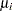, is related to the factors car and age for observationby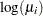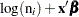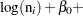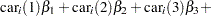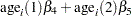The indicator variables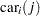is associated with theth level of the variable car for observationin the following way: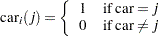A similar coding applies to age. The’s are parameters. The logarithm of the variable n is used as an offset—that is, a regression variable with a constant coefficient of 1 for each observation. Having the offset constant in the model is equivalent to fitting an expanded data set with 3000 observations, each with response variable y observed on an individual level. The log link is used to relate the mean and the factors car and age.

The following statements run PROC MCMC:

```proc mcmc data=insure outpost=insureout nmc=5000 propcov=quanew
maxtune=0 seed=7;
ods select PostSummaries PostIntervals;
parms alpha 0 beta_car1 0 beta_car2 0 beta_age 0;
prior alpha beta: ~ normal(0, prec = 1e-6);

mu = ln + alpha + beta_car1 * car_dummy1
+ beta_car2 * car_dummy2 + beta_age * age;
model c ~ poisson(exp(mu));
run;
```

The analysis uses a relatively flat prior on all the regression coefficients, with mean atand precision at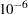. The option MAXTUNE=0 skips the tuning phase because the optimization routine (PROPCOV=QUANEW) provides good initial values and proposal covariance matrix.

There are four parameters in the model: alpha is the intercept; beta_car1 and beta_car2 are coefficients for the class variable car, which has three levels; and beta_age is the coefficient for age. The symbol mu connects the regression model and the Poisson mean by using the log link. The MODEL statement specifies a Poisson likelihood for the response variable c.

Posterior summary and interval statistics are shown in Output 52.3.8.

Output 52.3.8 MCMC Results
 Poisson Regression

The MCMC Procedure

Posterior Summaries
Parameter N Mean Standard
Deviation
Percentiles
25% 50% 75%
alpha 5000 -2.6403 0.1344 -2.7261 -2.6387 -2.5531
beta_car1 5000 -1.8335 0.2917 -2.0243 -1.8179 -1.6302
beta_car2 5000 -0.6931 0.1255 -0.7775 -0.6867 -0.6118
beta_age 5000 1.3151 0.1386 1.2153 1.3146 1.4094

Posterior Intervals
Parameter Alpha Equal-Tail Interval HPD Interval
alpha 0.050 -2.9201 -2.3837 -2.9133 -2.3831
beta_car1 0.050 -2.4579 -1.3036 -2.4692 -1.3336
beta_car2 0.050 -0.9462 -0.4498 -0.9485 -0.4589
beta_age 0.050 1.0442 1.5898 1.0387 1.5812

To fit the same model by using PROC GENMOD, you can do the following. Note that the default normal prior on the coefficientsis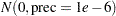, the same as used in the PROC MCMC. The following statements run PROC GENMOD and create Output 52.3.9:

```proc genmod data=insure;
ods select PostSummaries PostIntervals;
class car age(descending);
model c = car age / dist=poisson link=log offset=ln;
bayes seed=17 nmc=5000 coeffprior=normal;
run;
```

To compare, posterior summary and interval statistics from PROC GENMOD are reported in Output 52.3.9, and they are very similar to PROC MCMC results in Output 52.3.8.

Output 52.3.9 PROC GENMOD Results
 Poisson Regression

The GENMOD Procedure

Bayesian Analysis

Posterior Summaries
Parameter N Mean Standard
Deviation
Percentiles
25% 50% 75%
Intercept 5000 -2.6353 0.1299 -2.7243 -2.6312 -2.5455
carlarge 5000 -1.7996 0.2752 -1.9824 -1.7865 -1.6139
carmedium 5000 -0.6977 0.1269 -0.7845 -0.6970 -0.6141
age1 5000 1.3148 0.1348 1.2237 1.3138 1.4067

Posterior Intervals
Parameter Alpha Equal-Tail Interval HPD Interval
Intercept 0.050 -2.8952 -2.3867 -2.8755 -2.3730
carlarge 0.050 -2.3538 -1.2789 -2.3424 -1.2691
carmedium 0.050 -0.9494 -0.4487 -0.9317 -0.4337
age1 0.050 1.0521 1.5794 1.0624 1.5863

Note that the descending option in the CLASS statement reverses the sorting order of the class variable age so that the results agree with PROC MCMC. If this option is not used, the estimate for age has a reversed sign as compared to Output 52.3.9.Previous Page | Next Page | Top of Page